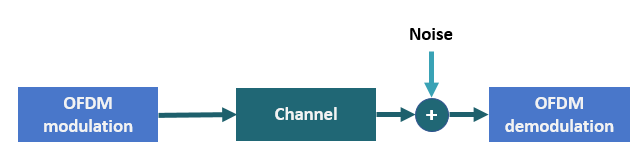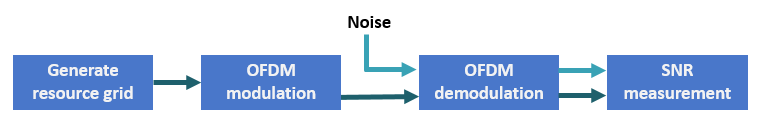# SNR Definition Used in Link Simulations

This example shows how 5G Toolbox™ link-level simulations define the signal-to-noise ratio (SNR).

### SNR Definition

5G Toolbox™ link examples (NR PDSCH Throughput and NR PUSCH Throughput) introduce AWGN to the received signal in the time domain, after the fading channel and before OFDM demodulation.The examples define the SNR as the average SNR per resource element (RE) per receive antenna. REs are defined in the resource grid (that is, in the frequency domain). To achieve the desired SNR, the examples introduce an equivalent noise level in the time domain.

The SNR is defined as

$\mathit{SNR}=\frac{{\mathit{S}}_{\mathrm{RE}}}{{\mathit{N}}_{\mathrm{RE}}}$.

${\mathit{S}}_{\mathrm{RE}}$ and ${\mathit{N}}_{\mathrm{RE}}$ are the average signal power per RE per receive antenna and the average noise power per RE per receive antenna, respectively. ${\mathit{N}}_{\mathrm{RE}}$ models the AWGN that is added to the signal.

For a signal $\mathit{x}$ with discrete Fourier transform (DFT) $\mathit{X}$, Parseval's theorem states

$\sum _{\mathit{n}=1}^{{\mathit{N}}_{\mathrm{FFT}}}{|{\mathit{x}}_{\mathit{n}}|}^{2}=\frac{1}{{\mathit{N}}_{\mathrm{FFT}}}\sum _{\mathit{k}=1}^{{\mathit{N}}_{\mathrm{FFT}}}{|{\mathit{X}}_{\mathit{k}}|}^{2}$.

${\mathit{N}}_{\mathrm{FFT}}$ is the FFT length. Divide the equation by ${\mathit{N}}_{\mathrm{FFT}}$ to get the average signal power

$\mathit{S}=\frac{1}{{\mathit{N}}_{\mathrm{FFT}}}\sum _{\mathit{n}=1}^{{\mathit{N}}_{\mathrm{FFT}}}{|{\mathit{x}}_{\mathit{n}}|}^{2}=\frac{1}{{\mathit{N}}_{\mathrm{FFT}}^{2}}\sum _{\mathit{k}=1}^{{\mathit{N}}_{\mathrm{FFT}}}{|{\mathit{X}}_{\mathit{k}}|}^{2}=\frac{1}{{\mathit{N}}_{\mathrm{FFT}}}{\mathit{X}}_{\mathrm{RMS}}^{2}$.

In 5G, the signal of interest does not use all FFT bins (or REs) because of guard bands or zero padding. Additionally, the signal allocation can occupy only a part of the available grid. If the signal uses only ${\mathit{K}}_{\mathit{S}}$ bins (or REs) of the FFT, the signal power is

$\mathit{S}=\frac{1}{{\mathit{N}}_{\mathrm{FFT}}}\sum _{\mathit{n}=1}^{{\mathit{N}}_{\mathrm{FFT}}}{|{\mathit{x}}_{\mathit{n}}|}^{2}=\frac{1}{{\mathit{N}}_{\mathrm{FFT}}^{2}}\sum _{\mathit{k}=1}^{{\mathit{K}}_{\mathit{S}}}{|{\mathit{X}}_{\mathit{k}}|}^{2}=\frac{{\mathit{K}}_{\mathit{S}}}{{{\mathit{N}}_{\mathrm{FFT}}}^{2}}{\mathit{X}}_{\mathrm{RMS}}^{2}$.

${\mathit{K}}_{\mathit{S}}$ is the number of nonzero power REs per OFDM symbol.

The signal power per RE is

${\mathit{S}}_{\mathrm{RE}}=\frac{\mathit{S}}{{\mathit{K}}_{\mathit{S}}}$.

The noise power per RE is

${\mathit{N}}_{\mathrm{RE}}=\frac{\mathit{N}}{{\mathit{N}}_{\mathrm{FFT}}}$.

Because the noise is added in the time domain, the noise occupies all bins, not just the allocated REs. Therefore, the noise power, $\mathit{N}$, is divided by ${\mathit{N}}_{\mathrm{FFT}}$ and not ${\mathit{K}}_{\mathit{S}}$.

Considering these definitions, the SNR becomes

$\mathit{SNR}=\frac{{\mathit{S}}_{\mathrm{RE}}}{{\mathit{N}}_{\mathrm{RE}}}=\frac{\frac{{\mathit{K}}_{\mathit{S}}}{{{{\mathit{K}}_{\mathit{S}}\mathit{N}}_{\mathrm{FFT}}}^{2}}{\mathit{X}}_{\mathrm{RMS}}^{2}}{\frac{\mathit{N}}{{\mathit{N}}_{\mathrm{FFT}}}}=\frac{{\mathit{X}}_{\mathrm{RMS}}^{2}}{{\mathit{N}}_{\mathrm{FFT}}\mathit{N}}$.

The 5G Toolbox link examples assume that ${\mathit{X}}_{\mathrm{RMS}}^{2}=1}{{\mathit{N}}_{\mathrm{Rx}}}$, where ${\mathit{N}}_{\mathrm{Rx}}$ is the number of receive antennas. This assumption means that the overall received power over all antennas is one.

`$\mathit{SNR}=\frac{1}{{\mathit{N}}_{\mathrm{Rx}}}}{{\mathit{N}}_{\mathrm{FFT}}\mathit{N}}=\frac{1}{{\mathit{N}}_{\mathrm{Rx}}{\mathit{N}}_{\mathrm{FFT}}\mathit{N}}$`

The noise power at the input of the OFDM demodulator is

$\mathit{N}=\frac{1}{{\mathit{N}}_{\mathrm{Rx}}{\mathit{N}}_{\mathrm{FFT}}\mathit{SNR}}$.

To generate noise with power $\mathit{N}$, scale the complex random samples by ${\mathit{N}}_{0}$.

`${\mathit{N}}_{0}=\sqrt{\frac{\mathit{N}}{2}}=\frac{1}{\sqrt{2{\mathit{N}}_{\mathrm{Rx}}{\mathit{N}}_{\mathrm{FFT}}\mathit{SNR}}}$`

The factor of 2 in this equation accounts for the complex nature of the noise samples.

Most 5G Toolbox examples that model a link use this scaling factor. This scaling factor assumes that the root mean squared of the signal RE values is ${\mathit{X}}_{\mathrm{RMS}}^{2}=1/{\mathit{N}}_{\mathrm{Rx}}$. This assumption does not always apply when using a propagation channel.

The propagation channel introduces a number of effects, such as correlation, antenna polarization, and antenna element gain. These channel effects can impact the signal power at the receiver. These effects make estimating the SNR at the receiver and setting up a simulation to model a specific SNR difficult. To overcome this difficulty, the SNR definition in the link examples does not consider any of the channel effects. The SNR definition matches the SNR that is measured without a fading channel and when ${\mathit{X}}_{\mathrm{RMS}}^{2}=1/{\mathit{N}}_{\mathrm{Rx}}$.

When you use a propagation channel (`nrTDLChannel` or `nrCDLChannel` object), take into account these considerations.

• Setting the `NormalizeChannelOutputs` property of the channel objects to `true` normalizes the channel outputs by the number of receive antennas such that ${\mathit{X}}_{\mathrm{RMS}}^{2}=1/{\mathit{N}}_{\mathrm{Rx}}$, as assumed in the derivation.

• Setting the `NormalizePathGains` property of the channel objects to `true` sets the total power of the average path gains to 0 dB.

• The SNR definition does not consider any of the channel effects.

### SNR Verification

This section verifies the equation that is derived in the previous section. Because the introduced SNR definition does not take into account any of the channel effects, this verification does not include a propagation channel.

This figure shows the setup to measure the SNR per RE per antenna.This setup implements these steps.

• Generate a resource grid with physical downlink shared channel (PDSCH) symbols.

• OFDM-modulate the grid.

• Generate AWGN.

• OFDM-demodulate the received signal and the noise separately.

• Measure the power of the signal and the noise per RE per antenna.

• Calculate and display the SNR.

Specify the desired SNR in dB.

```SNRdB = 0; rng("default") % Set default random number generator for repeatability```

Set the number of transmit and receive antennas. Because no channel exists, assume that the number of transmit and receive antennas is the same.

```nTxAnts = 2; nRxAnts = nTxAnts;```

Specify the carrier parameters.

```carrier = nrCarrierConfig; carrier.NSizeGrid = 52; % Grid size in resource blocks carrier.SubcarrierSpacing = 15; % Subcarrier spacing waveformInfo = nrOFDMInfo(carrier); % Waveform information pdsch = nrPDSCHConfig; pdsch.Modulation = "16QAM"; pdsch.PRBSet = 0:(carrier.NSizeGrid-1); % PDSCH allocation```

Create a norm-one precoding vector that is normalized by the number of layers.

`w = (1/sqrt(pdsch.NumLayers))*ones(pdsch.NumLayers,nTxAnts);`

To achieve the desired SNR, calculate the noise scaling factor: ${\mathit{N}}_{0}=\frac{1}{\sqrt{2{\mathit{N}}_{\mathrm{Rx}}{\mathit{N}}_{\mathrm{FFT}}\mathit{SNR}}}$.

```SNR = 10^(SNRdB/10); N0 = 1/sqrt(2.0*nRxAnts*double(waveformInfo.Nfft)*SNR);```

Generate precoded PDSCH symbols.

```[pdschIndices,pdschInfo] = nrPDSCHIndices(carrier,pdsch); pdschBits = randi([0 1],pdschInfo.G,1); pdschSymbols = nrPDSCH(carrier,pdsch,pdschBits); pdschSymbolsPrecoded = pdschSymbols*w;```

Create a resource grid and map the precoded PDSCH symbols to the resource grid.

```pdschGrid = nrResourceGrid(carrier,nTxAnts); [~,pdschAntIndices] = nrExtractResources(pdschIndices,pdschGrid); pdschGrid(pdschAntIndices) = pdschSymbolsPrecoded;```

OFDM-modulate.

`txWaveform = nrOFDMModulate(carrier,pdschGrid);`

Assume no channel exists. Because the SNR definition assumes that ${\mathit{X}}_{\mathrm{RMS}}^{2}=1/{\mathit{N}}_{\mathrm{Rx}}$, normalize the received signal by the number of receive antennas.

`rxWaveform = txWaveform/sqrt(nRxAnts);`

Generate AWGN.

`rxNoise = N0*complex(randn(size(rxWaveform)),randn(size(rxWaveform)));`

OFDM-demodulate the received signal (without noise), and extract the PDSCH symbols from the received grid to calculate ${\mathit{S}}_{\mathrm{RE}}$.

```% OFDM demodulation rxSignalGrid = nrOFDMDemodulate(carrier,rxWaveform); % PDSCH symbols extraction rxPDSCHSymbols = rxSignalGrid(pdschAntIndices);```

Measure the received signal power per RE, ${\mathit{S}}_{\mathrm{RE}}$, and the noise power per RE, ${\mathit{N}}_{\mathrm{RE}}$.

`${\mathit{S}}_{\mathrm{RE}}=\frac{\mathit{S}}{{\mathit{K}}_{\mathit{S}}}=\frac{{\mathit{K}}_{\mathit{S}}}{{\mathit{K}}_{\mathit{S}}{{\mathit{N}}_{\mathrm{FFT}}}^{2}}{\mathit{X}}_{\mathrm{RMS}}^{2}=\frac{1}{{{\mathit{N}}_{\mathrm{FFT}}}^{2}}{\mathit{X}}_{\mathrm{RMS}}^{2}$`

`${\mathit{N}}_{\mathrm{RE}}=\frac{\mathit{N}}{{\mathit{N}}_{\mathrm{FFT}}}$`

Verify that the measured SNR values approximate the specified SNR parameter.

```Sre = (1/waveformInfo.Nfft.^2)*rms(rxPDSCHSymbols).^2; Nre = (1/waveformInfo.Nfft)*rms(rxNoise).^2; for n=1:nRxAnts disp("Received signal power per RE antenna " + string(n) + " = " + string(pow2db(Sre(n))+30) + " dBm"); disp("Received noise power per RE antenna " + string(n) + " = " + string(pow2db(Nre(n))+30) + " dBm"); disp("SNR (antenna " + string(n) + ") = " + string(pow2db(Sre(n)/Nre(n))) + " dB"); end```
```Received signal power per RE antenna 1 = -33.186 dBm ```
```Received noise power per RE antenna 1 = -33.2432 dBm ```
```SNR (antenna 1) = 0.057195 dB ```
```Received signal power per RE antenna 2 = -33.186 dBm ```
```Received noise power per RE antenna 2 = -33.2371 dBm ```
```SNR (antenna 2) = 0.051146 dB ```